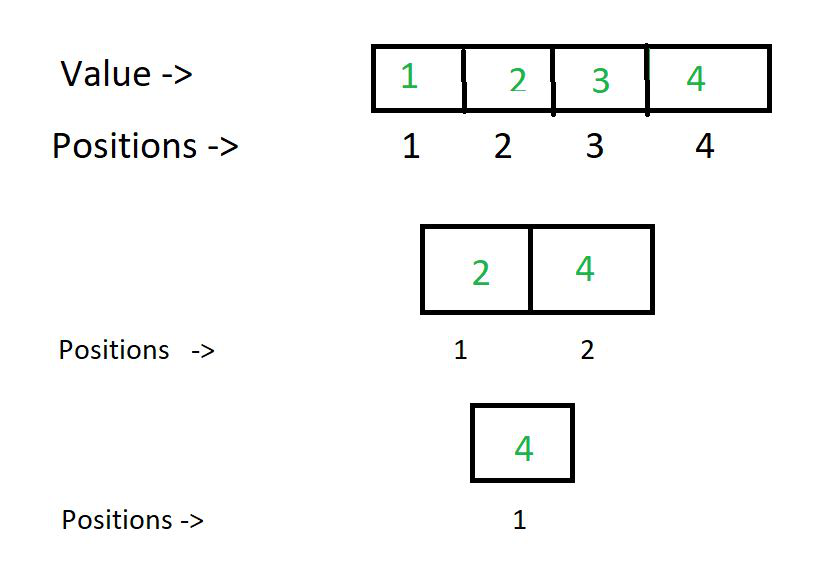Open in App
Not now

# Last remaining element after repeated removal of odd indexed elements

• Last Updated : 16 Aug, 2021

Given a positive integer N, the task is to print the last remaining element from a sequence [1, N] after repeatedly removing all the odd-indexed elements from the sequence.

Examples:

Input: N = 4
Output: 4
Explanation:Input: N = 9
Output: 8

Naive Approach: The naive approach to solve the above problem is to store the sequence. Iterate the sequence and remove the odd-indexed elements from it. Repeat the above steps till only one element remains.

Time Complexity: O(N logN)
Auxiliary Space: O(1)

Efficient Approach: Consider the below observation:

• In the first step, all the odd elements will be removed with a difference of 2
• In the second step, all even elements will be removed, starting from 2, with a difference 4
• In the second step, all even elements will be removed, starting from 4, with a difference 8
• Similarly, in the next step, all elements with difference 16 will be removed and so on.
• Therefore, in each step, you can see that the elements with difference 2X will be removed.
• In doing so, eventually all the elements will be removed except the largest power of 2 present in the sequence.

From the above observations, it can be deduced that:

last remaining element (L) = log2 N

Below is the implementation of the above approach.

## C++

 `#include ``#include``using` `namespace` `std;``int` `lastBlock(``int` `N)``{` `    ``// finding power of 2 less than or equal N``    ``int` `largestPower = log2(N);` `    ``// returning the last remaining number``    ``return` `pow``(2,largestPower);``}` `// Driver code``int` `main()``{` `    ``int` `N = 10;` `    ``cout << lastBlock(N);``    ``return` `0;``}` `// This code is contributed by maddler.`

## Java

 `// Java program for the above approach``import` `java.io.*;` `class` `GFG {` `  ``// Function to find last remaining element``  ``public` `static` `int` `lastBlock(``int` `N) {` `    ``// finding power of 2 less than or equal N``    ``int` `largestPower = (``int``)(Math.log(N) / Math.log(``2``));` `    ``// returning the last remaining number``    ``return` `(``int``)(Math.pow(``2``, largestPower));` `  ``}` `  ``// Driver code``  ``public` `static` `void` `main(String[] args)``  ``{``    ``int` `N = ``10``;` `    ``// Function Call``    ``System.out.println(lastBlock(N));``  ``}``}` `// This code is contributed by code_hunt.`

## Python3

 `# Python program for above approach.``import` `math` `# Function to find last remaining element``def` `lastBlock(N):` `    ``# finding power of 2 less than or equal N``    ``largestPower ``=` `(``int``)(math.log(N, ``2``))``    ` `    ``# returning the last remaining number``    ``return` `(``int``)(math.``pow``(``2``, largestPower))`  `# Driver Code``N ``=` `10` `# Function Call``print``(lastBlock(N))`

## C#

 `// C# program for the above approach``using` `System;` `class` `GFG {` `  ``// Function to find last remaining element``  ``public` `static` `int` `lastBlock(``int` `N) {` `    ``// finding power of 2 less than or equal N``    ``int` `largestPower = (``int``)(Math.Log(N) / Math.Log(2));` `    ``// returning the last remaining number``    ``return` `(``int``)(Math.Pow(2, largestPower));` `  ``}` `  ``// Driver code``  ``public` `static` `void` `Main(String[] args)``  ``{``    ``int` `N = 10;` `    ``// Function Call``    ``Console.Write(lastBlock(N));``  ``}``}` `// This code is contributed by shivanisinghss2110`

## Javascript

 ``

Output

`8`

Time Complexity: O(1)
Auxiliary Space: O(1)

My Personal Notes arrow_drop_up